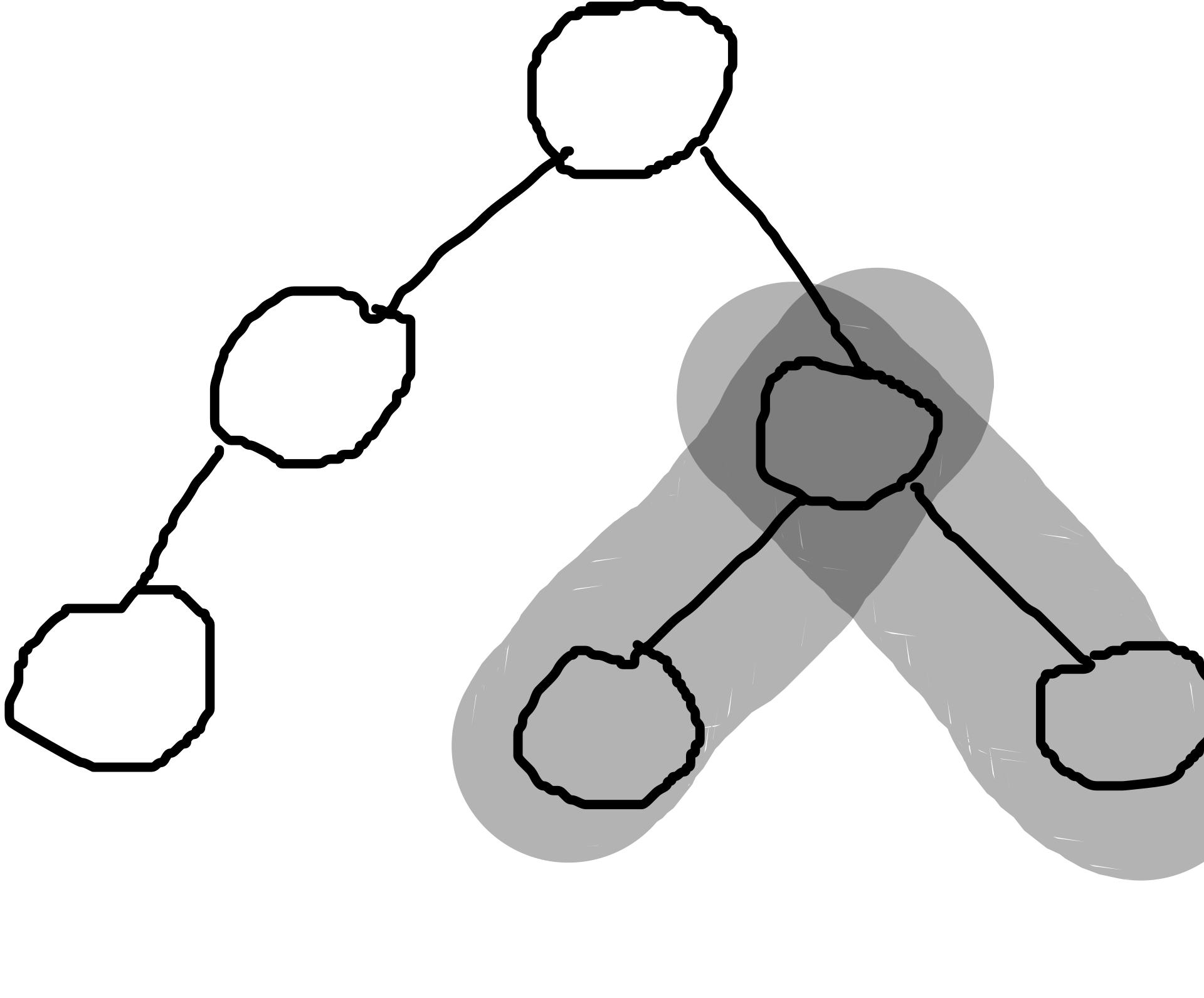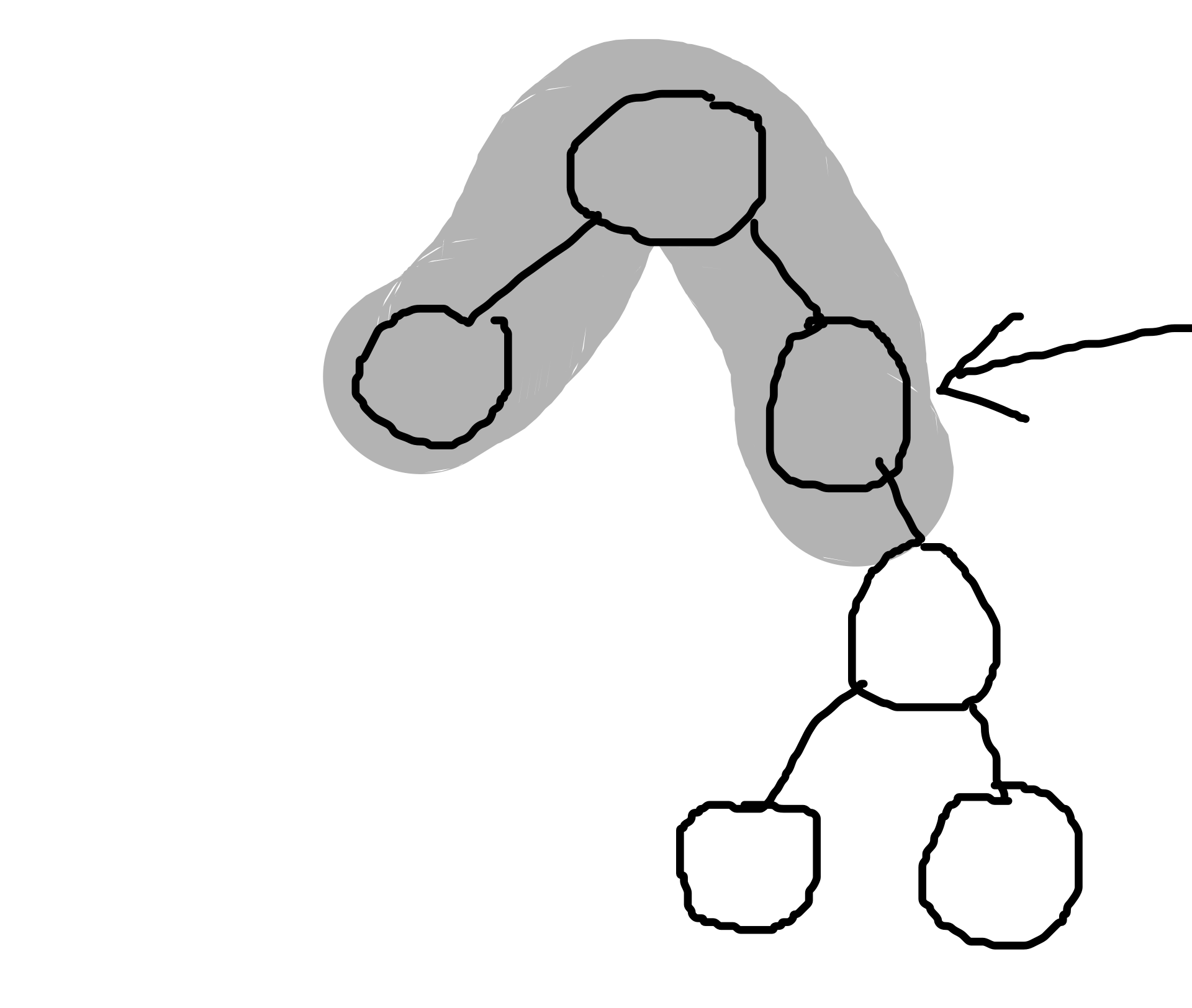# Closest leaf node to a given node in Binary Tree in C++

In this tutorial, we’ll see how to find the closest leaf to a node in a Binary tree in C++.

### Some Terminology:

Leaf node:

A leaf node in a binary tree is a node that has no child nodes.

Binary Tree:

A binary tree is a tree data structure with only 2 child nodes.

### Intuition:

To find the closest leaf to any node in a tree, there are only 2 possibilities  :

• Either the closest leaf is in the subtree from this node
• Or the closest leaf path goes through one of the ancestors of the nodeSo the sequence would go as follows:

• We first check what is the closest distance to a leaf in the subtree of the node.
• Then we go through all the parents in a loop till we reach the root and see the closest leaf to the subtree in the left part if the node is in the right subpart, or else the vice-a-versa.
• Then we compare these 2 distances obtained from the desired node’s subtree and the parent node and take the smallest one

Note: Here when we will compare the desired node subtree path to the parent node left/right subtree path there will be an additional factor of the length from parent to non-desired node subtree and the length of parent to the desired node subtree.

### Find closest leaf node to a given node in Binary Tree in C++

```#include <stdio.h>
#include <bits/stdc++.h>
using namespace std;

/*

My Node Data structure is a little special
As it stores whether the parent node of a node
Also it stores if it is present in the right or the left child of the parent node

*/

typedef struct Node{
struct Node* left;
struct Node* right;
struct Node* parent;
int value;

//Child Type = 'L' for left child | Child Type = 'R' for right child
char ChildType;
}Node;

//A function to create a NULL node
Node* CreateNode(int value)
{
Node* temp = new Node();
temp->value = value;
temp->left = temp->right = temp->parent = NULL;
return temp;
}

//A function to set the left child
void SetLeftChild(Node* root,int value)
{
root->left = new Node();
root->left->value = value;
root->left->ChildType = 'L';
root->left->parent = root;
}

//A function to set the right child
void SetRightChild(Node* root,int value)
{
root->right = new Node();
root->right->value = value;
root->right->ChildType = 'R';
root->right->parent = root;
}

//This function checks the Closest Leaf in the subtree of the node x and returns the value
//into ClosestDist
void ClosestLeafSubtree(Node* x,int lvl,int* ClosestDist)
{
if(x == NULL)
return;

//------If we get to a leaf node we check if the distance till this node----
//--------------------is the minimum till now or not-------------------------
if(x->left == NULL && x->right == NULL)
{
if(lvl < *ClosestDist)
*ClosestDist = lvl;
return;
}

//--------Recursively going through the left and right subtree-------
//-------------and increasing the level/path dist by 1----------------
ClosestLeafSubtree(x->left,lvl + 1,ClosestDist);
ClosestLeafSubtree(x->right,lvl + 1,ClosestDist);
}

void ClosestLeafDist(Node* x,int* closestDist)
{
//Setting a initial closest distance to be that from the subtree of the node
ClosestLeafSubtree(x,0,closestDist);

//cout<<*closestDist;

//This is distance from parent.[Initially,from the first parent = 1]
int dist_from_parent = 1;
//--------Creating a temp Node so as to not change the input node-------
Node* s = x;

//Looping through all the parents of the input node util we reach root
while(s->parent != NULL)
{
//If our input node is in the right subtree of the parent node
if(s->ChildType == 'R')
{
//Find the Closest Leaf in the left subtree of parent
int* temp_dist = new int(INT_MAX);
ClosestLeafSubtree(s->parent->left,0,temp_dist);

//----------------Check how our previous values compares to-----------------
// closestLeaf + (dist of leftsubtree to root = 1) + dist to desired node
if(*temp_dist+dist_from_parent+1<*closestDist)
*closestDist = *temp_dist+dist_from_parent+1;
}

//Else our input node is in the left subtree of the parent node
else
{
//Find the Closest Leaf in the right subtree of parent
int* temp_dist = new int(INT_MAX);
ClosestLeafSubtree(s->parent->right,0,temp_dist);

if(*temp_dist + dist_from_parent + 1<*closestDist)
*closestDist = *temp_dist + dist_from_parent + 1;
}

//We increase the distance from parent as we move to the previous ancestor
dist_from_parent += 1;
//We move to the previous ancestor
s = s->parent;

}

}
int main(void) {
Node* root = CreateNode(1);

SetLeftChild(root,12);
SetRightChild(root,13);

SetLeftChild(root->right,13);
SetLeftChild(root->right->left,13);
SetLeftChild(root->right->left->left,13);
SetRightChild(root->right,13);

SetRightChild(root->right->right,13);
SetRightChild(root->right->right->right,13);
SetRightChild(root->right->right->right->right,13);
SetRightChild(root->right->right->right->right->right,13);

int* closestDist = new int(INT_MAX);
ClosestLeafDist(root->right->right,closestDist);
cout<<*closestDist;

return 0;
}
``````Output:

3```